#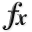N-D Test Functions K¶

class go_benchmark.Katsuura(dimensions=2)

Katsuura test objective function.

This class defines the Katsuura global optimization problem. This is a multimodal minimization problem defined as follows: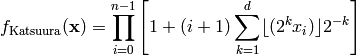Where, in this exercise,.

Here,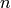represents the number of dimensions and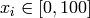for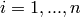.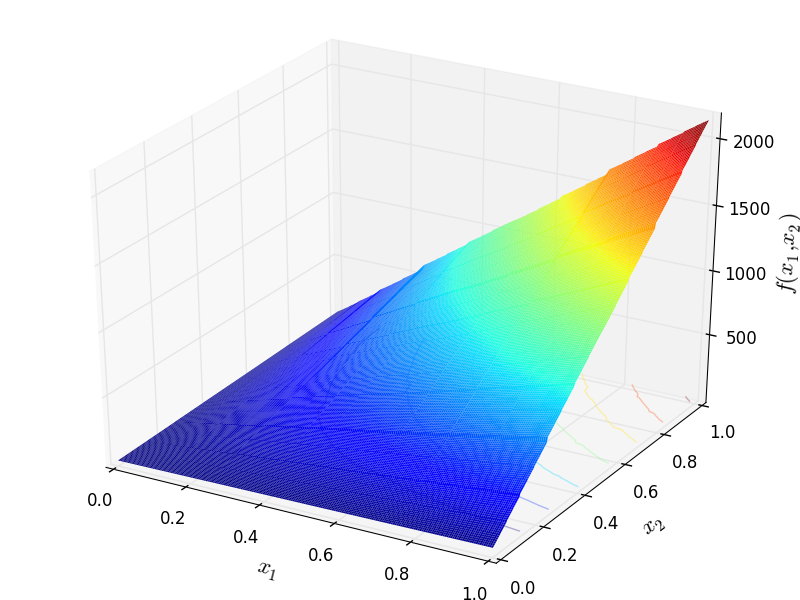Two-dimensional Katsuura function

Global optimum: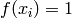for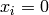for.

class go_benchmark.Keane(dimensions=2)

Keane test objective function.

This class defines the Keane global optimization problem. This is a multimodal minimization problem defined as follows: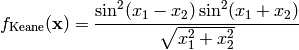Here,represents the number of dimensions and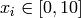for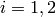.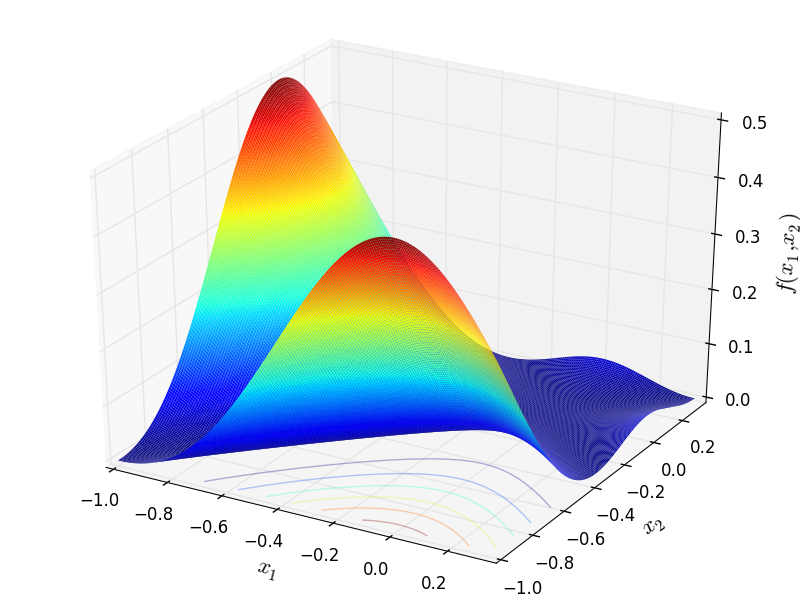Two-dimensional Keane function

Global optimum:for.

class go_benchmark.Kowalik(dimensions=4)

Kowalik test objective function.

This class defines the Kowalik global optimization problem. This is a multimodal minimization problem defined as follows: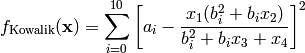Where:Here,represents the number of dimensions andfor.

Global optimum:for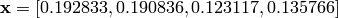.

#### Previous topic

N-D Test Functions J

#### Next topic

N-D Test Functions L